# 9.6: Other Useful Distributions

$$\newcommand{\vecs}{\overset { \rightharpoonup} {\mathbf{#1}} }$$ $$\newcommand{\vecd}{\overset{-\!-\!\rightharpoonup}{\vphantom{a}\smash {#1}}}$$$$\newcommand{\id}{\mathrm{id}}$$ $$\newcommand{\Span}{\mathrm{span}}$$ $$\newcommand{\kernel}{\mathrm{null}\,}$$ $$\newcommand{\range}{\mathrm{range}\,}$$ $$\newcommand{\RealPart}{\mathrm{Re}}$$ $$\newcommand{\ImaginaryPart}{\mathrm{Im}}$$ $$\newcommand{\Argument}{\mathrm{Arg}}$$ $$\newcommand{\norm}{\| #1 \|}$$ $$\newcommand{\inner}{\langle #1, #2 \rangle}$$ $$\newcommand{\Span}{\mathrm{span}}$$ $$\newcommand{\id}{\mathrm{id}}$$ $$\newcommand{\Span}{\mathrm{span}}$$ $$\newcommand{\kernel}{\mathrm{null}\,}$$ $$\newcommand{\range}{\mathrm{range}\,}$$ $$\newcommand{\RealPart}{\mathrm{Re}}$$ $$\newcommand{\ImaginaryPart}{\mathrm{Im}}$$ $$\newcommand{\Argument}{\mathrm{Arg}}$$ $$\newcommand{\norm}{\| #1 \|}$$ $$\newcommand{\inner}{\langle #1, #2 \rangle}$$ $$\newcommand{\Span}{\mathrm{span}}$$

The normal distribution is the distribution that statistics makes most use of (for reasons to be discussed shortly), and the binomial distribution is a very useful one for lots of purposes. But the world of statistics is filled with probability distributions, some of which we’ll run into in passing. In particular, the three that will appear in this book are the t distribution, the χ2 distribution and the F distribution. I won’t give formulas for any of these, or talk about them in too much detail, but I will show you some pictures.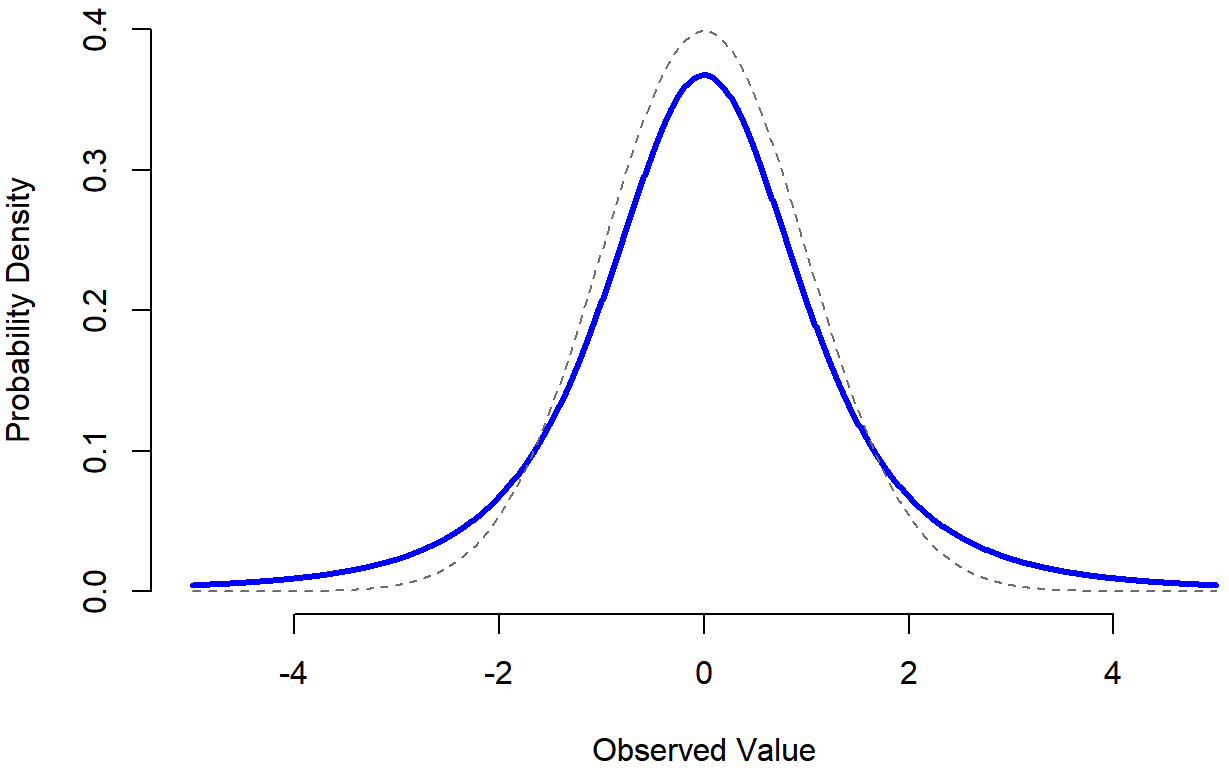Figure 9.13: A t distribution with 3 degrees of freedom (solid line). It looks similar to a normal distribution, but it’s not quite the same. For comparison purposes, I’ve plotted a standard normal distribution as the dashed line. Note that the “tails” of the t distribution are “heavier” (i.e., extend further outwards) than the tails of the normal distribution? That’s the important difference between the two.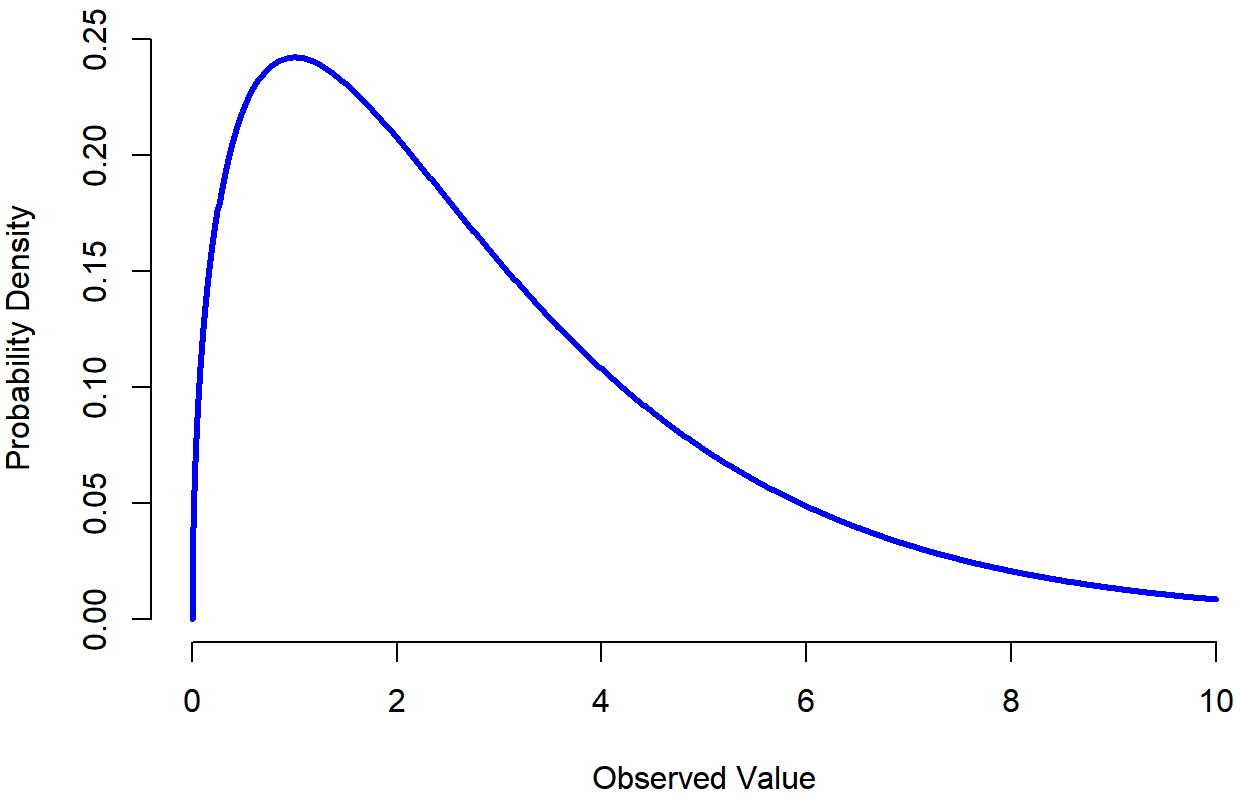Figure 9.14: A chi2 distribution with 3 degrees of freedom. Notice that the observed values must always be greater than zero, and that the distribution is pretty skewed. These are the key features of a chi-square distribution.Figure 9.15: An F distribution with 3 and 5 degrees of freedom. Qualitatively speaking, it looks pretty similar to a chi-square distribution, but they’re not quite the same in general.
• The t distribution is a continuous distribution that looks very similar to a normal distribution, but has heavier tails: see Figure 9.13. This distribution tends to arise in situations where you think that the data actually follow a normal distribution, but you don’t know the mean or standard deviation. As you might expect, the relevant R functions are dt(), pt(), qt() and rt(), and we’ll run into this distribution again in Chapter 13.
• The χ2 distribution is another distribution that turns up in lots of different places. The situation in which we’ll see it is when doing categorical data analysis (Chapter 12), but it’s one of those things that actually pops up all over the place. When you dig into the maths (and who doesn’t love doing that?), it turns out that the main reason why the χ2 distribution turns up all over the place is that, if you have a bunch of variables that are normally distributed, square their values and then add them up (a procedure referred to as taking a “sum of squares”), this sum has a χ2 distribution. You’d be amazed how often this fact turns out to be useful. Anyway, here’s what a χ2 distribution looks like: Figure 9.14. Once again, the R commands for this one are pretty predictable: dchisq(), pchisq(), qchisq(), rchisq().
• The F distribution looks a bit like a χ2 distribution, and it arises whenever you need to compare two χ2 distributions to one another. Admittedly, this doesn’t exactly sound like something that any sane person would want to do, but it turns out to be very important in real world data analysis. Remember when I said that χ2 turns out to be the key distribution when we’re taking a “sum of squares”? Well, what that means is if you want to compare two different “sums of squares”, you’re probably talking about something that has an F distribution. Of course, as yet I still haven’t given you an example of anything that involves a sum of squares, but I will… in Chapter 14. And that’s where we’ll run into the F distribution. Oh, and here’s a picture: Figure 9.15. And of course we can get R to do things with F distributions just by using the commands df(), pf(), qf() and rf().

Because these distributions are all tightly related to the normal distribution and to each other, and because they are will turn out to be the important distributions when doing inferential statistics later in this book, I think it’s useful to do a little demonstration using R, just to “convince ourselves” that these distributions really are related to each other in the way that they’re supposed to be. First, we’ll use the rnorm() function to generate 1000 normally-distributed observations:

normal.a <- rnorm( n=1000, mean=0, sd=1 )
print(head(normal.a))

##  -0.4728528 -0.4483396 -0.5134192  2.1540478 -0.5104661  0.3013308

So the normal.a variable contains 1000 numbers that are normally distributed, and have mean 0 and standard deviation 1, and the actual print out of these numbers goes on for rather a long time. Note that, because the default parameters of the rnorm() function are mean=0 and sd=1, I could have shortened the command to rnorm( n=1000 ). In any case, what we can do is use the hist() function to draw a histogram of the data, like so:

hist( normal.a )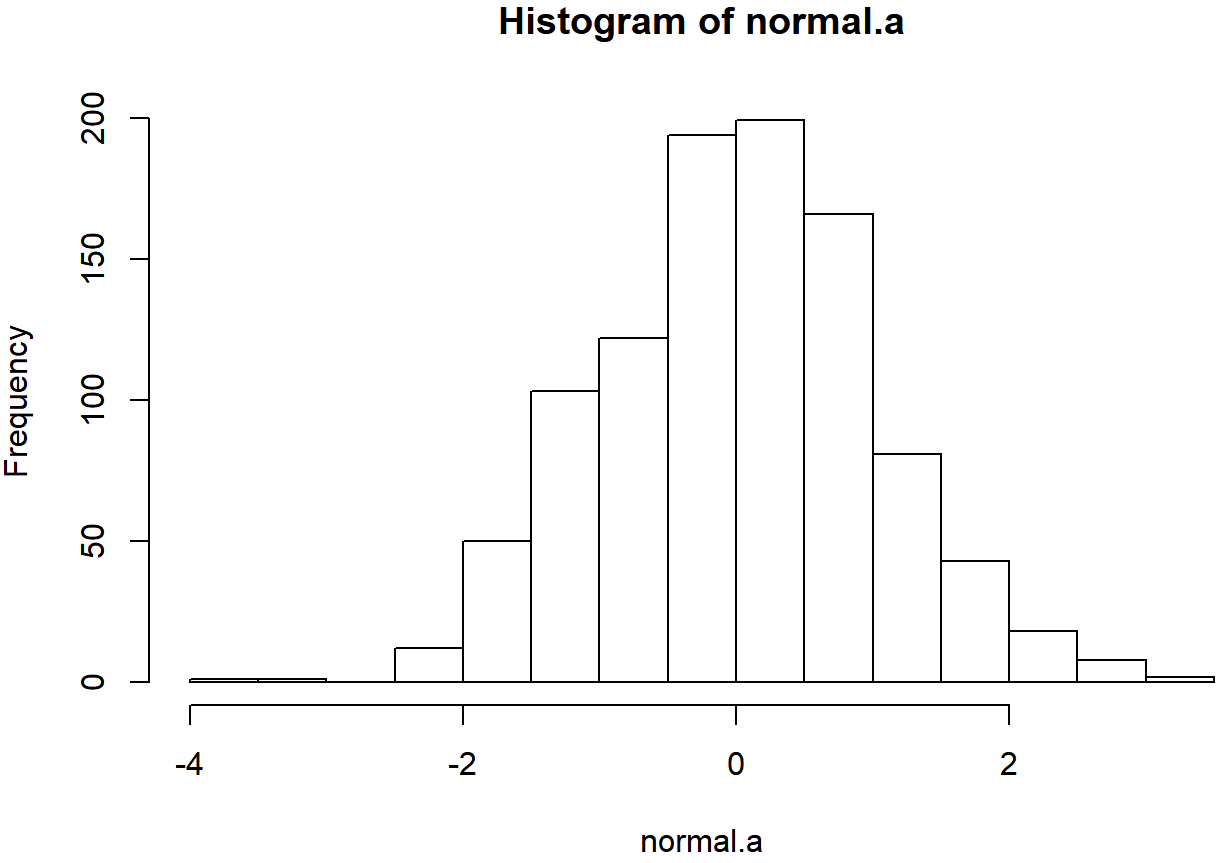If you do this, you should see something similar to Figure ??. Your plot won’t look quite as pretty as the one in the figure, of course, because I’ve played around with all the formatting (see Chapter 6), and I’ve also plotted the true distribution of the data as a solid black line (i.e., a normal distribution with mean 0 and standard deviation 1) so that you can compare the data that we just generated to the true distribution.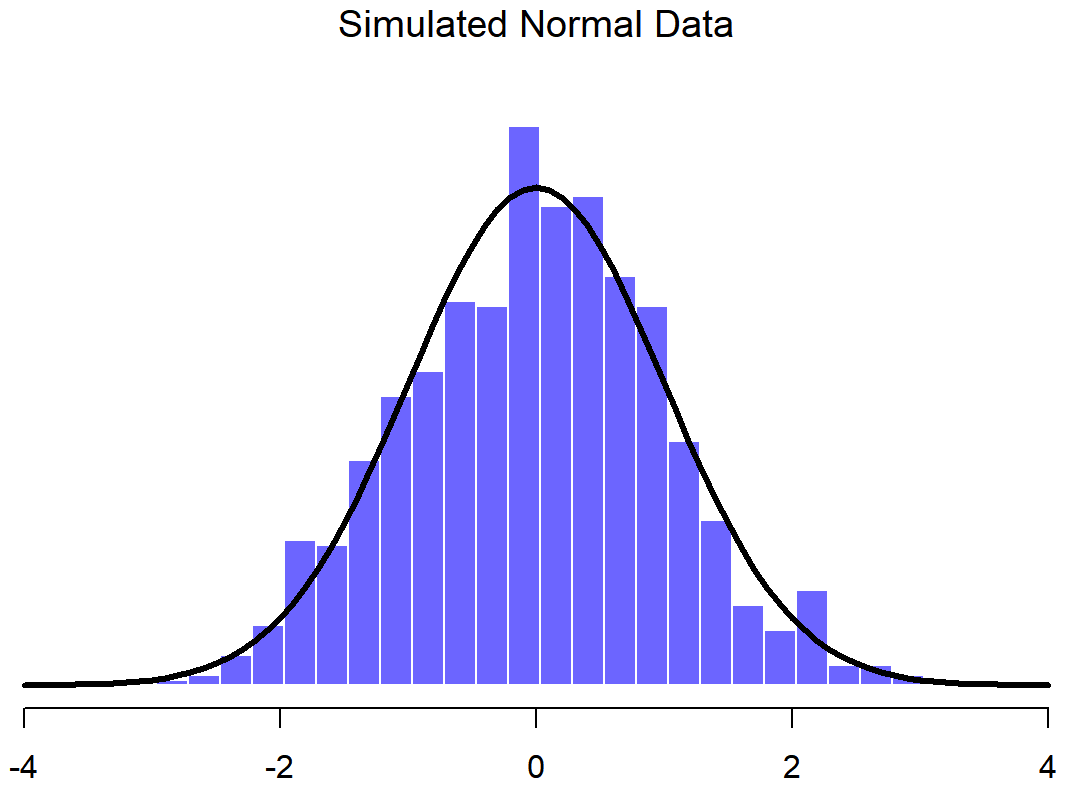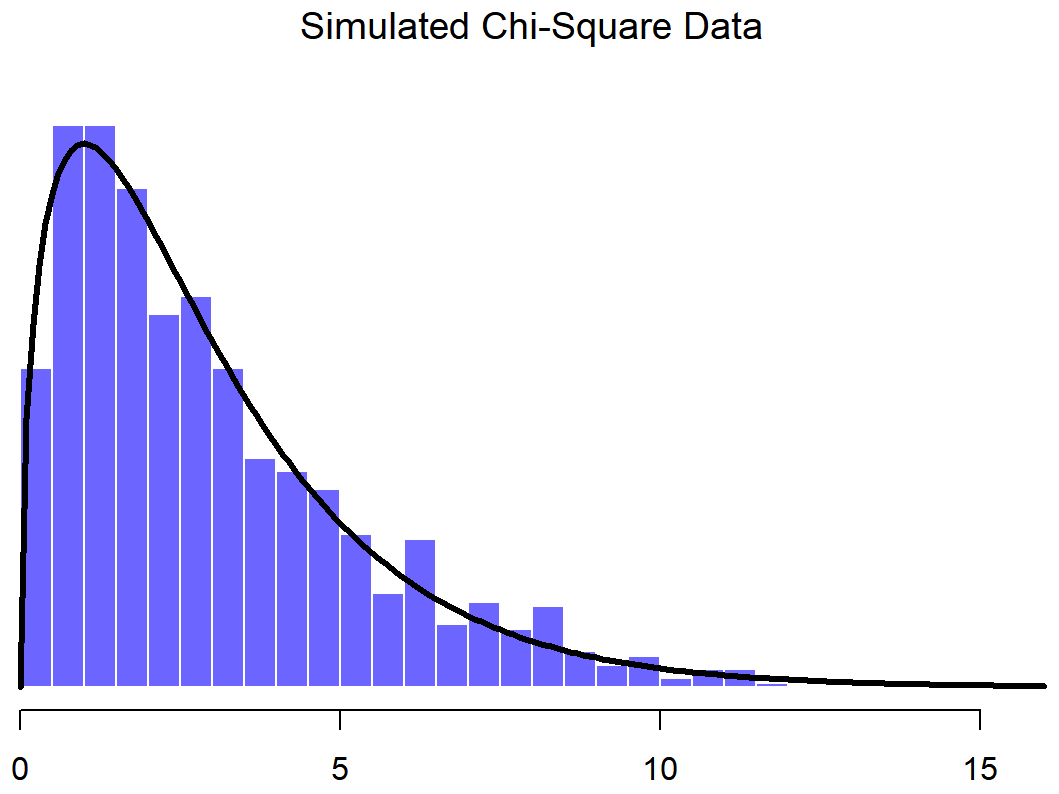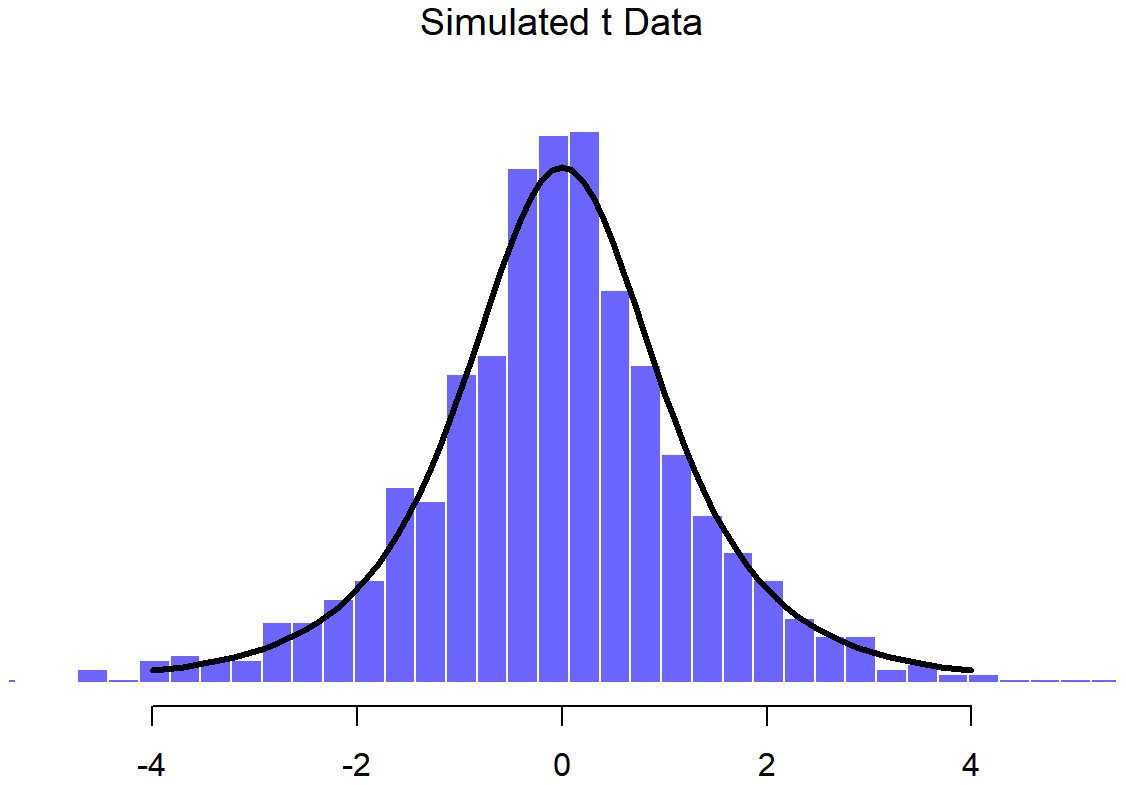In the previous example all I did was generate lots of normally distributed observations using rnorm() and then compared those to the true probability distribution in the figure (using dnorm() to generate the black line in the figure, but I didn’t show the commmands for that). Now let’s try something trickier. We’ll try to generate some observations that follow a chi-square distribution with 3 degrees of freedom, but instead of using rchisq(), we’ll start with variables that are normally distributed, and see if we can exploit the known relationships between normal and chi-square distributions to do the work. As I mentioned earlier, a chi-square distribution with k degrees of freedom is what you get when you take k normally-distributed variables (with mean 0 and standard deviation 1), square them, and add them up. Since we want a chi-square distribution with 3 degrees of freedom, we’ll need to supplement our normal.a data with two more sets of normally-distributed observations, imaginatively named normal.b and normal.c:

normal.b <- rnorm( n=1000 )  # another set of normally distributed data
normal.c <- rnorm( n=1000 )  # and another!

Now that we’ve done that, the theory says we should square these and add them together, like this

chi.sq.3 <- (normal.a)^2 + (normal.b)^2 + (normal.c)^2

and the resulting chi.sq.3 variable should contain 1000 observations that follow a chi-square distribution with 3 degrees of freedom. You can use the hist() function to have a look at these observations yourself, using a command like this,

hist( chi.sq.3 )and you should obtain a result that looks pretty similar to the chi-square plot in Figure ??. Once again, the plot that I’ve drawn is a little fancier: in addition to the histogram of chi.sq.3, I’ve also plotted a chi-square distribution with 3 degrees of freedom. It’s pretty clear that – even though I used rnorm() to do all the work rather than rchisq() – the observations stored in the chi.sq.3 variable really do follow a chi-square distribution. Admittedly, this probably doesn’t seem all that interesting right now, but later on when we start encountering the chi-square distribution in Chapter 12, it will be useful to understand the fact that these distributions are related to one another.

We can extend this demonstration to the t distribution and the F distribution. Earlier, I implied that the t distribution is related to the normal distribution when the standard deviation is unknown. That’s certainly true, and that’s the what we’ll see later on in Chapter 13, but there’s a somewhat more precise relationship between the normal, chi-square and t distributions. Suppose we “scale” our chi-square data by dividing it by the degrees of freedom, like so

scaled.chi.sq.3 <- chi.sq.3 / 3

We then take a set of normally distributed variables and divide them by (the square root of) our scaled chi-square variable which had df=3, and the result is a t distribution with 3 degrees of freedom:

normal.d <- rnorm( n=1000 )  # yet another set of normally distributed data
t.3 <- normal.d / sqrt( scaled.chi.sq.3 )  # divide by square root of scaled chi-square to get t

If we plot the histogram of t.3, we end up with something that looks very similar to the t distribution in Figure ??. Similarly, we can obtain an F distribution by taking the ratio between two scaled chi-square distributions. Suppose, for instance, we wanted to generate data from an F distribution with 3 and 20 degrees of freedom. We could do this using df(), but we could also do the same thing by generating two chi-square variables, one with 3 degrees of freedom, and the other with 20 degrees of freedom. As the example with chi.sq.3 illustrates, we can actually do this using rnorm() if we really want to, but this time I’ll take a short cut:

chi.sq.20 <- rchisq( 1000, 20)  # generate chi square data with df = 20...
scaled.chi.sq.20 <- chi.sq.20 / 20  # scale the chi square variable...
F.3.20 <-  scaled.chi.sq.3  / scaled.chi.sq.20 # take the ratio of the two chi squares...
hist( F.3.20 ) # ... and draw a pictureThe resulting F.3.20 variable does in fact store variables that follow an F distribution with 3 and 20 degrees of freedom. This is illustrated in Figure ??, which plots the histgram of the observations stored in F.3.20 against the true F distribution with df1=3 and df2=20. Again, they match.

Okay, time to wrap this section up. We’ve seen three new distributions: χ2, t and F. They’re all continuous distributions, and they’re all closely related to the normal distribution. I’ve talked a little bit about the precise nature of this relationship, and shown you some R commands that illustrate this relationship. The key thing for our purposes, however, is not that you have a deep understanding of all these different distributions, nor that you remember the precise relationships between them. The main thing is that you grasp the basic idea that these distributions are all deeply related to one another, and to the normal distribution. Later on in this book, we’re going to run into data that are normally distributed, or at least assumed to be normally distributed. What I want you to understand right now is that, if you make the assumption that your data are normally distributed, you shouldn’t be surprised to see χ2, t and F distributions popping up all over the place when you start trying to do your data analysis.

This page titled 9.6: Other Useful Distributions is shared under a CC BY-SA 4.0 license and was authored, remixed, and/or curated by Danielle Navarro via source content that was edited to the style and standards of the LibreTexts platform; a detailed edit history is available upon request.